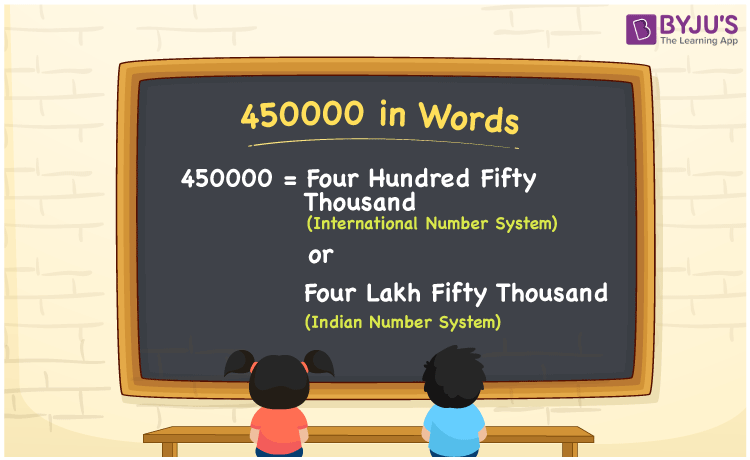# 450000 in Words

We can write 450000 in words as Four Hundred Fifty Thousand (in International Number System)or Four Lakh Fifty Thousand (in Indian Number System).If you have saved Rs. 450000 in 9 months, you can write “I have saved Rupees Four Lakh Fifty Thousand in nine months”. We know that 450000 is a cardinal number as it shows a specific quantity. Learn how to convert 450000 to words with the help of the place value chart here.

 450000 in words International Number System Indian Number System Four Hundred Fifty Thousand Four Lakh Fifty Thousand Four Hundred Fifty Thousand or Four Lakh Fifty Thousand in numerical form 450000

## 450000 in English Words

English is an important language to learn how to write numbers in words. Therefore, students are advised to learn English which is the most widely used language in the Education field. Hence, 450000 in words is written as Four Hundred Fifty Thousand or Four Lakh Fifty Thousand.## How to Write 450000 in Words?

It is essential to determine the place value of each digit of the given number to write the number name of 450000. The below chart represents the place value of the given number.

 Lakhs Ten-Thousands Thousands Hundreds Tens Ones 4 5 0 0 0 0

Therefore, we can write the expanded form as:

4 x Lakh + 5 x Ten-Thousand + 0 x Thousand + 0 x Hundred + 0 × Ten + 0 × One

= 4 x 100000 + 5 x 10000 + 0 x 1000 + 0 x 100 + 0 x 10 + 0 x 1

= 400000 + 50000 + 0 + 0 + 0 + 0

= 400000 + 50000

= 450000

= Four Hundred Fifty Thousand or Four Lakh Fifty Thousand

Thus, 450000 in words is written as Four Hundred Fifty Thousand or Four Lakh Fifty Thousand.

Interesting way of writing 450000 in words

4 = Four

45 = Forty-Five

450 = Four Hundred and Fifty

4500 = Four Thousand Five Hundred

45000 = Forty-Five Thousand

450000 = Four Hundred Fifty Thousand or Four Lakh Fifty Thousand

Hence, the word form of the number 450000 is Four Hundred Fifty Thousand or Four Lakh Fifty Thousand.

450000 is a natural number that is succeeded by 449999 and preceded by 450001.

• 450000 in words – Four Hundred Fifty Thousand or Four Lakh Fifty Thousand
• Is 450000 an odd number? – No
• Is 450000 an even number? – Yes
• Is 450000 a perfect square number? – No
• Is 450000 a perfect cube number? – No
• Is 450000 a prime number? – No
• Is 450000 a composite number? – Yes

## Frequently Asked Questions on 450000 in Words

Q1

### Write 450000 in words.

We can represent 450000 in words as Four Hundred Fifty Thousand or Four Lakh Fifty Thousand.
Q2

### Is the number 450000 an odd number?

No, 450000 is not an odd number.
Q3

### Express the value 450500 minus 500 and write the answer in words.

450500 minus 500 = 450500 – 500 = 450000 450000 in words is written as Four Hundred Fifty Thousand or Four Lakh Fifty Thousand.